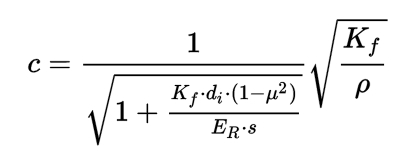# Adblue: urea water solution

Hey,

I am currently making a master thesis concerning water hammer on an adblue network.

But I require the fluid properties of this urea water solution.

Thank you very much,

P. Fédor

Hi @fplatteau,

do you intend do make a CFD simulation to achieve this or what do you have in mind?

Best,

Jousef

Yes,
That is correct. But I require the bulk modulus of the urea water solution.

Greetings,

F. Platteau

Hi again @fplatteau,

I am not an expert in this but could you not just use this formula:where

Rho = Density of the fluid [kg/m³],
K_f = Bulk Modulus of the fluid [N/m²],
E_R = Young’s Modulus of the pipe [N/m²],
d_i = Inner diameter of the pipe [m],
s = Thickness of the pipe [m]
mu = Poisson’s ratio of the material

Assuming that the Bulk Modulus is the unknown you could simply rearrange the formula, can’t you?

Tell me if I am mistaken.

Source of the formula: Druckstoß - Wikipedia

Best,

Jousef

Hey Jousefm,

Thank You very much for the information. But to use the formula, I require the wave celerity before I can calculate the Bulk modulus. I found on the internet by searching on topics related to modelling of SCR techniques with adblue, a paper with a graph containing the information required.

So I would like to thank you for your help and interest.

Yours sincerely,

Platteau F.

Hi @fplatteau,

Do you have another approach for this then? I also found a few papers about these SCR techniques. What was the information that was missing? Just the celerity then?

Cheers,

Jousef